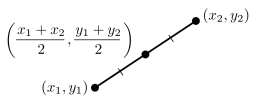# Number 6911

On the number axis, point P is the image of the number -2.54, and point Q is the image of the number 10.71. Find which number is the point R such that Q is the center of the line PR.

R =  23.96

### Step-by-step explanation:Did you find an error or inaccuracy? Feel free to write us. Thank you!

Tips for related online calculators
Looking for help with calculating arithmetic mean?
Looking for a statistical calculator?

#### Grade of the word problem:

We encourage you to watch this tutorial video on this math problem: